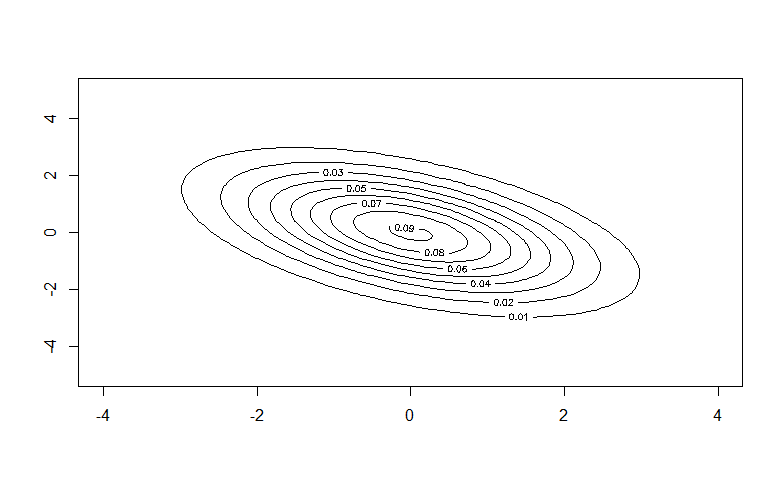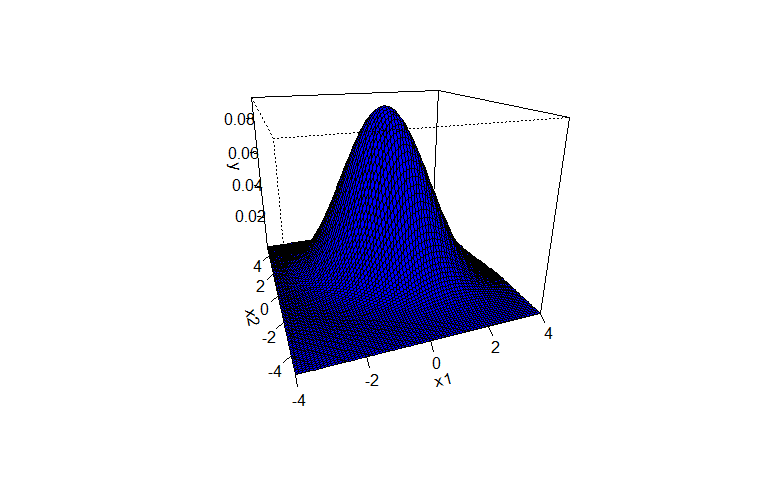# Visualizing the Bivariate Gaussian Distribution in R

• Last Updated : 03 May, 2022

The Gaussian distribution (better known as the normal distribution) is one of the most fundamental probability distributions in statistics. A bivariate Gaussian distribution consists of two independent random variables. One can notice a bell curve while visualizing a bivariate gaussian distribution. Two random variables X1 and X2 are bivariate normal if aX1+bX2 has a normal distribution for all a, b ∈ R.

## Probability Distribution Function (PDF) of a bivariate gaussian distribution

The density function describes the relative likelihood of a random variable X at a given sample. Mathematically the PDF of two variables X and Y in bivariate Gaussian distribution is given by:where,

•• μ = mean
• σ = standard deviation
• ρ = correlation of x1 and x2

If P = 2 then this is a bivariate gaussian distribution.

## Visualizing the Bivariate Gaussian Distribution in R

We will visualize bivariate Gaussian distribution in R by plotting them using the functions from the mnormt() package.

install.packages('mnormt')

We will use dmnorm( ) to simulate a normal distribution.

dmnorm( ): mnorm(x, mean = rep(0, d), varcov, log = FALSE)

Now, we will use the contour( ) function to create a contour plot, to get a 2-D visualization of the bivariate gaussian distribution

## R

 library(mnormt)set.seed(0)x1 <- seq(-4, 4, 0.1)x2 <- seq(-5, 5, 0.1)mean <- c(0, 0)cov <- matrix(c(2, -1, -1, 2), nrow=2)f <- function(x1, x2) dmnorm(cbind(x1, x2), mean, cov)y <- outer(x1, x2, f) # create contour plotcontour(x1, x2, y)

n : sample size.
mean : mean of each variable.
cov : covariance matrix of the two variables.

Output:For 3-D visualization of the distribution, we will create a surface plot using persp( ) function of the package.

persp(x = seq(0, 1, length.out = nrow(z)),y = seq(0, 1, length.out = ncol(z)),z, xlim = range(x), ylim = range(y),zlim = range(z, na.rm = TRUE),xlab = NULL, ylab = NULL, zlab = NULL,main = NULL, sub = NULL,theta = 0, phi = 15, r = sqrt(3), d = 1,scale = TRUE, expand = 1,col = “white”, border = NULL, theta = -135, lphi = 0,shade = NA, box = TRUE, axes = TRUE, nticks = 5,ticktype = “simple”, …)

## R

 install.packages('mnormt')library(mnormt) set.seed(0)x1 <- seq(-4, 4, 0.1)x2 <- seq(-5, 5, 0.1)mean <- c(0, 0)cov <- matrix(c(2, -1, -1, 2), nrow=2)f <- function(x1, x2) dmnorm(cbind(x1, x2), mean, cov)y <- outer(x1, x2, f) #create surface plotpersp(x1, x2, y, theta=-20, phi=20, col = 'blue',      expand=0.8, ticktype='detailed')

Output:My Personal Notes arrow_drop_up